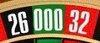trizeroyouroul.com79 716

## step tree

```                                       1. R
+               -
+               -
2. R            3. R
+            -              +               -
++          +-              -+              --
4. R        5. R            6. R            7. R
+       -       +       -       +       -       +       -
+++     ++-     +-+     +--     -++     -+-     --+     ---
8. R    9. R    10.R    11.R    12.R    13.R    14.R    15.R
+   -   +   -   +   -   +   -   +   -   +   -   +   -   +   -
++++    ++-+    +-++    +--+    -+++    -+-+    --++    ---+
+++-    ++--    +-+-    +---    -++-    -+--    --+-    ----
___________________________________________________________________
Amount  Red  4   3   3   2   3   2   2   1   3   2   2   1   2   1   1   0
16. 17. 17. 18. 17. 18. 18. 19. 17. 18. 18. 19. 18. 19. 19. 20.
16. R 4    17. R 3    18. R 2    19. R 1    20. R 0
```

+ always means a win, - a loss
in the line below is the sequence of wins and losses.
RBRB is equal to +-+- here.

Annotation:
This step tree is build 1:1 logical.
One could have achieved the same result by merging "equal steps":
+-- is "1" like -+- or --+
or even: +- is equal to -+

## roulette simulator

As this explanation is for demonstration of the process of a strategy in the roulette simulator,
no simplification was made. The result would have been the same.
If the order of wins and losses shall be considered too, no simplification can be made.

The flowcharts illustrate the step tree very well:
Relativity dot-flowchart: